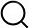# Also in the Article

Scaling under constant average degree

Constructing graphs from genetic encodings
Sci Rep, Jun 24, 2021;

Procedure

An important limit considered in graph models is the scaling of network metrics with system size, when a constant average degree is maintained. Specifically, how does the number of rules r(N) scale with system size $N=2b$ given a hand-imposed scaling of the average degree $⟨k⟩$?

Solving for the number of rules given the network density $ρ=1-1-22(x-b)2r$ and average degree $⟨k⟩=ρN$ gives

In the limit of $b>>1$, using $log(1+a)≈a$, () is simplified to

If, in addition, $b-x=g$ is constant, then the number of rules scales as

In this limit, therefore, the number of rules scales inversely with system size. This can be understood based on the fact that wiring rule sizes, in terms of the number of new edges they introduce, grow proportionally to the square of the system size N.

If, on the other hand, $b-x>>1$ (such as when x/b or x are kept constant), then

In this regime, the number of rules always scales as the system size N.

Q&A